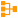# Case

 Block Group: Logic Icon:The Case block evaluates a list of conditions and returns the result the corresponds to the first condition that is met.

You can use the Case block to evaluate a source value against a series of test values. The Case block compares the source value with each test value in sequence, until the first true expression is created. Then, the Case block returns the value that corresponds to this expression.

An expression will evaluate to FALSE if the data type of the test value is not comparable to the data type of the source value.

For information on using dataflow blocks, see Dataflow.

## Video Tutorial: Case Block

More video tutorials are here.

## Input/Output Properties

The following properties of the Case block can take input and give output.

• op (enum)
• input
• case n
• then n
• else

op specifies the arithmetic, comparison, or text operator to apply to the source value and each test value. It is used in conjunction with input and case n to form an expression that can be evaluated to TRUE or FALSE.

input specifies the source value that is to be compared with each test value.

case n specifies a test value to compare with the input.

then n specifies the result value if the expression formed by input, operator, and case n is TRUE.

else specifies the result value to return if none of the expressions evaluates to TRUE.

## Output Property

The following property of the Case block can give output but cannot take input.

• output

output returns the then n value for the first case n value that creates a true expression. If none of the expressions is true, output returns the else value.

## How to Make a Latch

You can make a latch by binding the output property of a Case block to the else property. In a latch, the output changes only if one of the expressions evaluates to TRUE.

## Examples

The following image demonstrates three examples of the Case block. The leftmost Case block returns “Heating” if input 0 is below 65, then “Off” if input 0 is below 85, and then “Cooling” otherwise. The middle Case block returns the name of a time of day that the block determines from a time string. The rightmost Case block is a latch. The latch’s output only changes if its input becomes A or B.